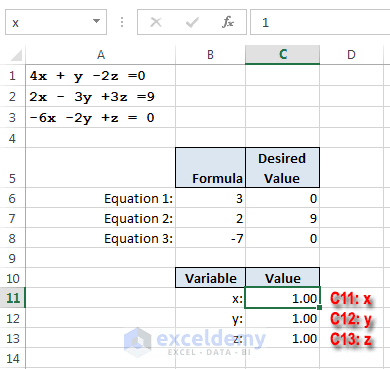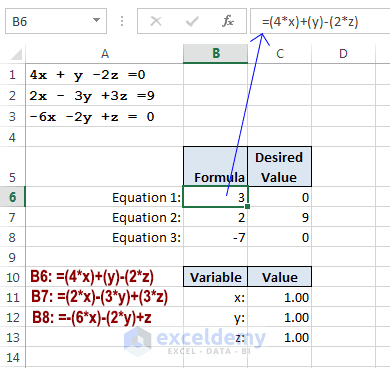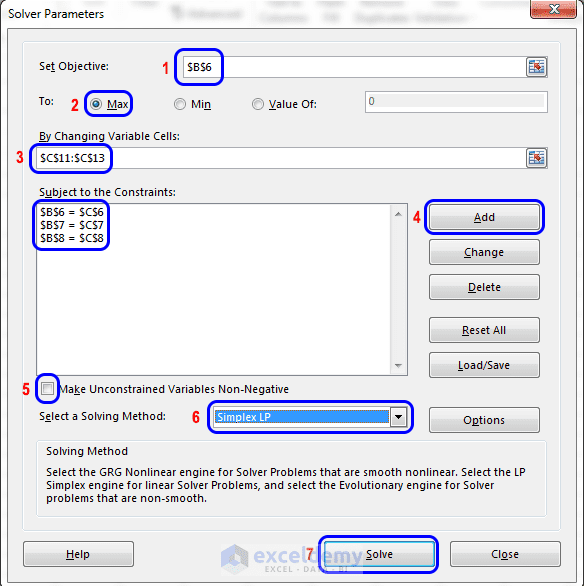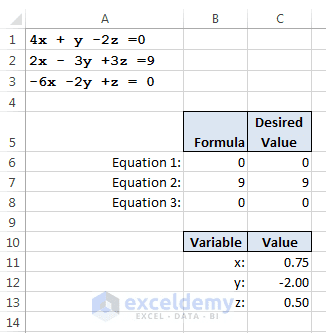Disclosure: This post may contain affiliate links, meaning when you click the links and make a purchase, we receive a commission.

# How to solve simultaneous linear equations in Excel using Solver

In this blog post, I will show you how to solve simultaneous linear equations in Excel using solver add-in. You can also use Excel Matrix functions (MMULT, MINVERSE) to solve linear equations. That is really easy. But in this article, we shall focus only on the Solver method.

Let’s start.

We’re going to explain how to solve a set of three linear equations with three variables.

Here’s an example of a set of three linear equations:

4x+ y– 2z= 0
2x– 3y+ 3z= 9
–6x– 2y+ z= 0

We shall let Solver answer this question: What are the values of x, y, and z that satisfy all three equations?

The following figure shows a workbook set up to solve this problem. To make the formulas more readable, we have given names to three cells in this workbook:

• x: C11
• y: C12
• z: C13

All the three named cells are initialized to 1.0 (which certainly aren’t the solutions of the equations).The solver will attempt to solve this series of linear equations.

The three equations are represented by formulas in the cell range B6:B8:

• B6: =(4*x)+(y)-(2*z)
• B7: =(2*x)-(3*y)+(3*z)
• B8: =-(6*x)-(2*y)+(z)B6, B7 & B8 are holding these formulas.

These formulas use x, y, and z named cells’ values. The desired result for these three formulas is contained in the cell range C6:C8.

The solver will adjust the values in x, y, and z— that is, the changing cells in C11:C13 — subject to these constraints:

B6=C6
B7=C7
B8=C8

Read More: What is Solver in Excel

## Solving Simultaneous Linear Equation Step by Step

Now use the following steps to set up the Solver Parameters dialog box. This dialog box appears when you choose Data ➪ Analysis ➪ Solver.

### Step 1:

Fill Set Objective field with this value: \$B\$6.

### Step 2:

Select radio button of the Max option in To control.

### Step 3:

Select cell \$C\$11 to \$C\$13 to fill the field By Changing Variable Cells. This field will show then \$C\$11: \$C\$13.

### Step 4:

Add constraints one by one. The constraints are \$B\$6=\$C\$6, \$B\$7=\$C\$7, and \$B\$8=\$C\$8. These constraints will be shown in the Subject to the Constraints field. Click this article to know more about adding constraints.Step by step process done in the Solver Parameters dialog box.

### Step 5:

Deselect the Make Unconstrained Variables Non-Negative check box.

### Step 6:

Select Simplex LP from the Select a Solving Method drop-down list.

### Step 7:

Now click the Solve button. You will find Solver Results dialog box shown in the following figure. Click OK, you are done with your result.‘Solver Results’ dialog box.

Note: This problem doesn’t have a target cell because it’s not trying to maximize or minimize anything. However, the Solver Parameters dialog box insists that you specify a formula for the Set Objective field. Therefore, just enter a reference to any cell that has a formula.

The following figure shows the solution. The x(0.75), y(–2.0), and z(0.5) values satisfy all three equations.Solver solved the simultaneous equations.

Note: A set of linear equations may have one solution, no solution, or an infinite number of solutions.
Master Data Analysis: Top Data Analysis Courses Online

Happy Excelling 🙂

Hello!
Welcome to my Excel blog! It took me some time to be a fan of Excel. But now I am a die-hard fan of MS Excel. I learn new ways of doing things with Excel and share here. Not only how to guide on Excel, but you will get also topics on Finance, Statistics, Data Analysis, and BI. Stay tuned!

1. ReplyBala Apr 6, 2016 at 12:19 PM

Hi,
I am trying to solve linear equations with multiple unknowns. Sample below. Problem is I have only one equation with multiple unknows
19.6=a+0.0124x+0.0304y+0.175z
I need a excel function to give possible values for the variables in above equation. Anyone knows how to do it?

P.S: I am not looking for solutions where we have three unknowns and hence we cant solve with less than three questions. I need to know what are the possible (not unique) values that satisfies the above equation

Thanks

2. ReplyRangarajan Dec 29, 2017 at 8:34 AM

Need more lessons on solving quadratic and linear equations.

3. ReplyJ Daigle Apr 26, 2018 at 2:32 AM

4. Reply• Reply